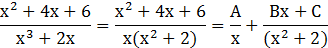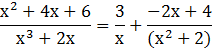### Sample Problem

Write the partial fraction decomposition of.

#### SolutionMultiply both sides by x(x2+2) [the lowest common denominator]

x2+4x+6=A(x2+2)+(Bx+C)x

x2+4x+6=Ax2+2A+Bx2+Cx

x2+4x+6=(A+B)x2+Cx+2AA=3, C=4

Substituting A=3 into the first equation à 3+B=1 à B=-2

So, the partial fraction decomposition is: